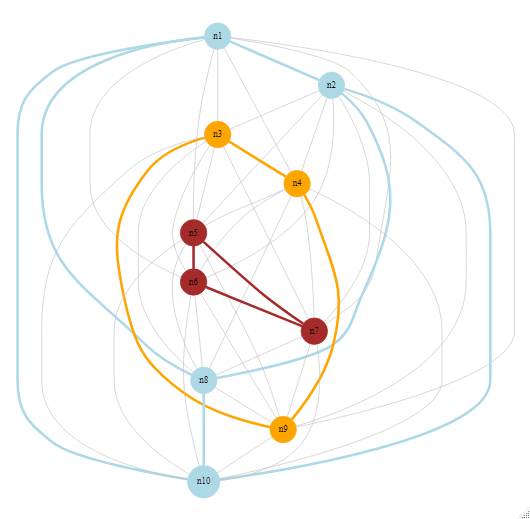## Monday, January 31, 2022

### Is a heuristic a solver?

Can we call a heuristic (especially a meta-heuristic) a solver? I am not so sure.

Warning: this is somewhat of an opinionated rant. Many of you may not agree.

The name "solver" seems to imply that the underlying algorithm knows when an optimization problem is solved. By that I mean: it can stop at some stage and report "solved". Meta-heuristics typically don't have a clue about optimality, and just keep on churning until hitting some form of time or iteration limit. (One could devise some stopping criteria basic on stochastic reasoning, but that is not often used in meta-heuristics).

So my proposal is:

Heuristics (including meta-heuristics) should not be called solvers. They don't know when a problem is solved.

## Tuesday, January 25, 2022

### A different coloring problem

In  the following problem is proposed:

• Consider a complete (undirected) graph,
• where each arc has a weight.
• Given a collection of colors, color each node,
• such that the sum of the weights of arcs with same-colored nodes is minimized.
• We can assume some sparsity in the weights.

The user expects the number of nodes to be between 20 and 50, and there are just a small number of colors.Minimize weights for the colored arcs

The picture shows a complete graph (all nodes have a direct arc to each other) with 10 nodes. The optimal coloring of nodes minimizes the sum of weights along the colored edges. An edge is colored if the two incident nodes have the same color.

## Sunday, January 23, 2022

### Coloring the US county map

A famous result in graph theory is that we can color a planar graph with just four colors . The MIP model for coloring a graph is really simple. Let's try this with a relatively large data set: the map of US counties. We'll see that there are some hurdles on the way.

#### Building block: MIP model for coloring a graph

The problem is as follows: we want to assign a color to each node such that nodes connected by an arc don't have the same color. The objective is to minimize the number of different colors. For the MIP model we use an assignment variable:  $\color{darkred}x_{n,c} = \begin{cases}1 & \text{if node n gets color c} \\ 0 & \text{otherwise}\end{cases}$ To keep track of the colors we have: $\color{darkred}u_c = \begin{cases}1 & \text{color c is used for some nodes} \\ 0 & \text{otherwise}\end{cases}$

The MIP model can look like:

MIP Model
\begin{align} \min & \sum_c \color{darkred}u_c \\ & \sum_c \color{darkred}x_{n,c}=1 &&\forall n \\ & \color{darkred}x_{i,c}+\color{darkred}x_{j,c} \le \color{darkred}u_c && \forall \color{darkblue}{\mathit{arc}}_{i,j}, c \\ & \color{darkred}u_c \le \color{darkred}u_{c-1} && \forall c\gt 1 \\ & \color{darkred}x_{n,c} \in \{0,1\} \\ & \color{darkred}u_c \in \{0,1\} \end{align}

## Wednesday, January 19, 2022

### An All-Paths Network Problem

In  a graph problem is proposed:

• Given an undirected network,
• Find all paths between two nodes,
• Such that no arc is used more than once,
• And the path does not contain more than $$M$$ arcs
Notice that nodes can be visited several times. I thought this could be a good job for a solution pool approach.

#### Preliminaries

As I am more familiar with directed graphs, let's start with setting up a small random, sparse, directed graph.

I start with 10 nodes and say there is a 20% probability that a link exists between two nodes, $$i \rightarrow j$$. So the data set looks like:

 ----     30 SET n  nodes  n1 ,    n2 ,    n3 ,    n4 ,    n5 ,    n6 ,    n7 ,    n8 ,    n9 ,    n10  ----     30 SET a  directed arcs             n1          n2          n3          n4          n5          n6 n1                     YES                     YESn2                                                                     YESn3                                 YESn4                                 YES         YES                     YESn6                                                         YESn7         YES                                             YESn8                                 YESn9         YES  +          n7          n8          n9         n10 n1                     YESn2                     YESn3                     YES         YESn4         YESn5                                 YESn6                                             YESn8                                             YESn9         YES                                 YES

Using $$n_1$$ as the start node and $$n_{10}$$ as the end node, we can visualize this as: